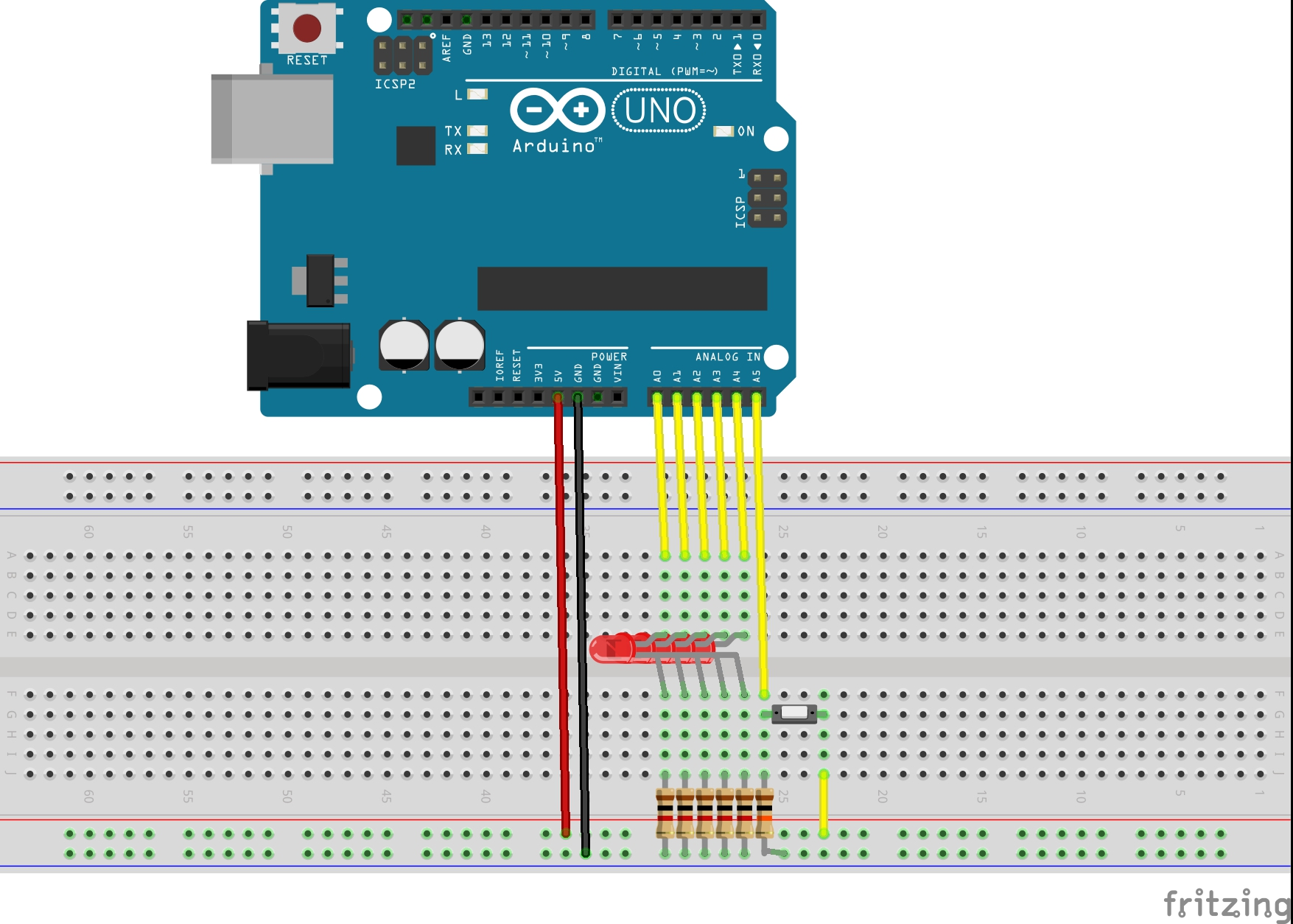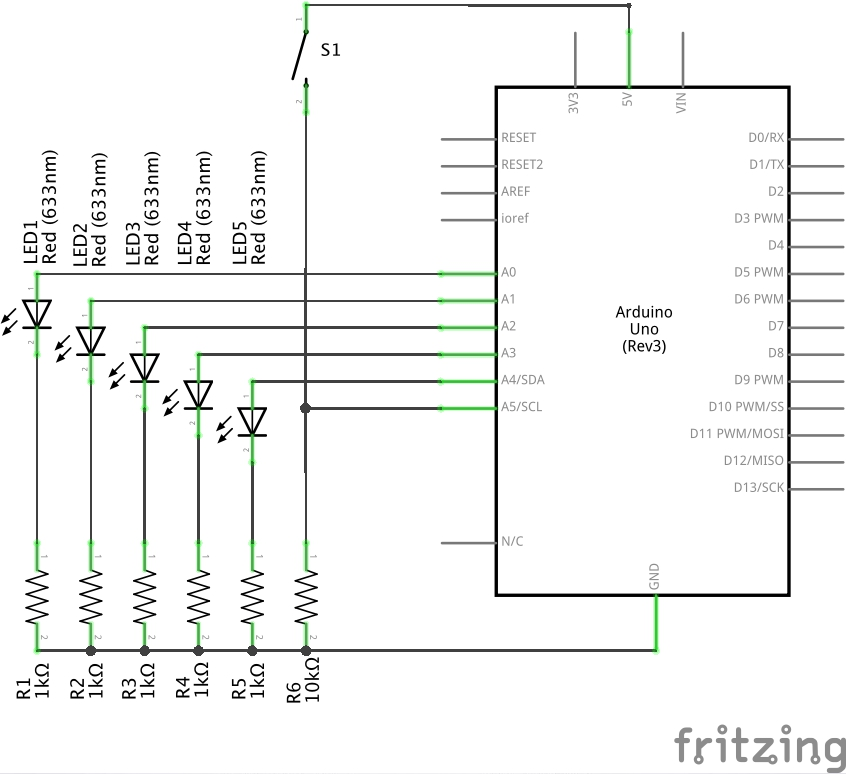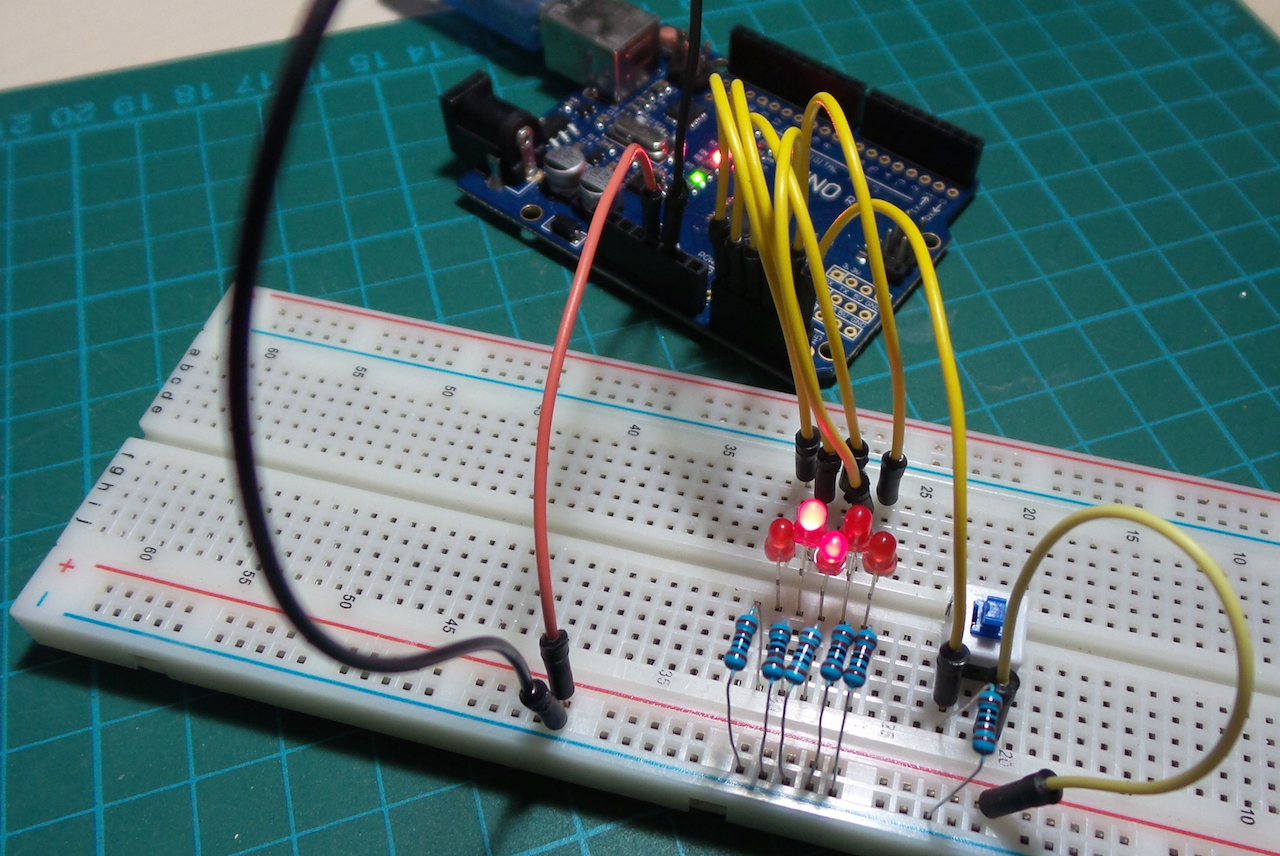# #240 DigitalInputWithAnalogPins

Demonstrate how analog pins can also be used as a digital GPIO

This is a simple demonstration of a tip shared by peter@txplore.com in the Arduino Tips and Tricks email newsletter

On the Arduino Uno, there are 6 analog pins. While we’d normally use AnalogRead to read A/D values and AnalogWrite to write PWM, they can also be used as GPIO digital pins.

The conventional analog pin mnemonics can be used:

``````pinmode(A0, INPUT);
``````

If desired it’s also possible to use the raw pin number (if driving pins algorithmically for example). Pin definitions are found in the `pins_arduino.h`. For the Uno, the

To use them as digital inputs, just use this reference table:

Analog Pin Pin pins_arduino.h definition
0 14 static const uint8_t A0 = 14;
1 15 static const uint8_t A1 = 15;
2 16 static const uint8_t A2 = 16;
3 17 static const uint8_t A3 = 17;
4 18 static const uint8_t A4 = 18;
5 19 static const uint8_t A5 = 19;

Using the pin number explicitly:

``````pinmode(14, INPUT);
``````

### Example

The DigitalInputWithAnalogPins.ino sketch is a simple exercise of analog pins A0..A5 using both digital input and output.

Five pins A0..A4 are set to sequentially drive corresponding LEDs at about 5mA.

A push-button is read on A5. Pressing the button reverse the LED sequence.

## Construction